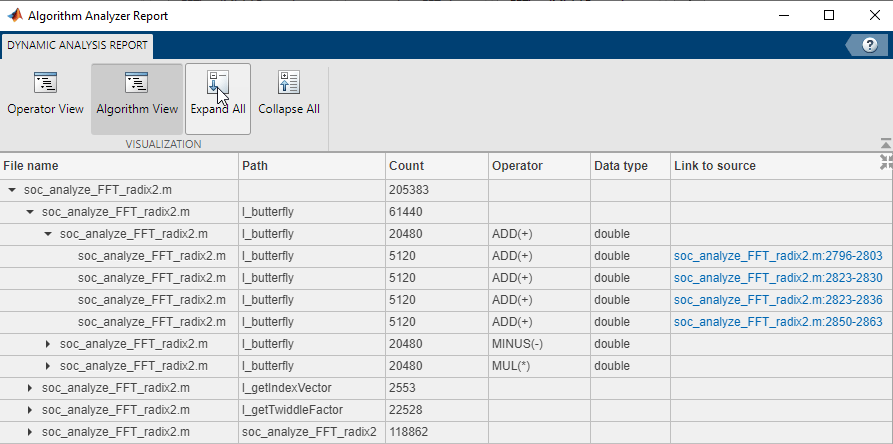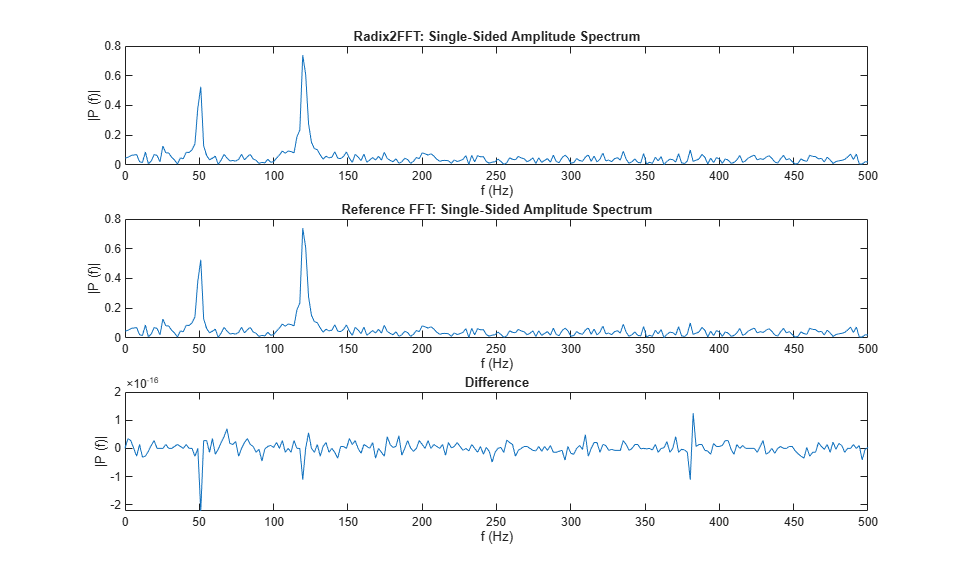# Estimate Number of Operators for MATLAB Algorithm

This example shows how to estimate the number of arithmetic operators in an algorithm written in MATLAB®. Analyze a radix 2 FFT algorithm and generate reports showing operator usage.

### Radix 2 FFT Algorithm and Testbench

Analyze the number of arithmetic operators in the `soc_analyze_FFT_radix2` function. Calculate the number of arithmetic operators used during the execution of the function. The testbench `soc_analyze_fft_tb` provides stimulus and verifies the implementation of the radix 2 FFT algorithm (`soc_analyze_FFT_radix2`) against the MATLAB FFT function (`fft`) .

Open the `soc_analyze_FFT_tb.m` file in the MATLAB editor to examine the structure of the testbench.

```open soc_analyze_FFT_tb ```

The testbench generates a test signal with two sinusoids, one at 50 Hz with an amplitude of 0.7 and another at 120 Hz with an amplitude of 1. The signal has a sampling frequency of 1 kHz with additive random noise. The testbench calculates the FFT output for the above test signal for the FFT-length specified as the `FFTLen` argument. The testbench compares the output of the function to the output of the MATLAB FFT function (`fft`) and plots the results.

### Generate Operator-Count Reports for 1024 Points Radix 2 FFT

To estimate the number of operators for the radix 2 FFT, use the `socFunctionAnalyzer` function, and provide the testbench function `soc_analyze_FFT_tb` as an argument. By default, the function generates reports for all the functions called from within the testbench function and lists all the operators used.

To generate a report for only the algorithm (`soc_analyze_FFT_radix2`), and not for the testbench, use the `'RestrictFunction'` name-value pair argument with the value `'soc_analyze_FFT_radix2.m'`. Use the `'RestrictOperator'` name-value pair argument to filter the report and show only three operators by setting its value to `{'ADD','MINUS','MUL'}`. Set the `'OutputFolder'` name-value pair argument to specify a folder location for generated reports.

Execute this command to generate reports for a simulation of a 1024-points radix 2 FFT algorithm. The command simulates the design while counting operators and generating a report.

```socFunctionAnalyzer('soc_analyze_FFT_tb.m','FunctionInputs',1024, ... 'Folder','report_1024','IncludeFunction','soc_analyze_FFT_radix2.m', ... 'IncludeOperator',{'ADD','MINUS','MUL'}); ```
```Generating operators analysis report for /tmp/Bdoc22a_1891349_205291/tp8c57dad1/ex65369380/soc_analyze_FFT_tb.m ... Saving report files in /tmp/Bdoc22a_1891349_205291/tp8c57dad1/ex65369380/report_1024. Operator estimate: <a href="matlab: socAlgorithmAnalyzerReport('/tmp/Bdoc22a_1891349_205291/tp8c57dad1/ex65369380/report_1024/soc_analyze_FFT_tb.mat')">Open report viewer</a> Done. ```Observe the radix 2 FFT algorithm and the reference MATLAB FFT function (`fft`) results in the simulation plot above. Verify that those results are very similar and the difference between those results is in order of 10e-12.

### Analyze Operator Estimation Report

Open the report by clicking the Open report viewer link on the MATLAB console. Alternatively, you can use the `socAlgorithmAnalyzerReport` function. The report provides two views. The first view is the operator view, which presents the data such that each row corresponds to an operator. To use this view, click Operator View on the report toolstrip. The second view is the algorithm view, where each row corresponds to a MATLAB function. To use this view, click Algorithm View on the report toolstrip.

By default, the report opens with the operator view. The report opens the aggregate view of each operator and data type. For example, for a 1024 points radix 2 FFT there are a total of 91,649 additions [ADD(+)] of data type `double` and 67,094 subtractions [MINUS(-)] of data type `int32`. To get the detailed report for each operator, expand that operator. The report shows the operator count as used in various functions. For example, the butterfly function `l_butterfly` contains four `double` additions that executed 5,120 times each. Trace the operator by clicking on one of the links in the last column of the report to highlight the location of the operator in the `soc_analyze_FFT_radix2` file.Switch to the algorithm view, by clicking the Algorithm View button. Expand the report and view the operator counts for all the functions under the file `soc_analyze_FFT_radix2.m`. You can view the counts of each operator with their data types by expanding another level. You can also use the Expand All and Collapse All buttons on the report toolstrip to navigate the report. To trace a specific operator to the MATLAB code, click the corresponding link in the column Link to source in the report.### Generate Reports for 512 Points Radix 2 FFT

To observe the correlation between the number of operations and the number of points in the FFT, compare the previous report with the one for a 512 points radix 2 FFT. Generate reports for a 512 points radix 2 FFT by passing a value of 512 to the `'FunctionInputs'` name-value pair argument as in this command.

``` socFunctionAnalyzer('soc_analyze_FFT_tb.m','FunctionInputs',512, ... 'Folder','report_512','IncludeFunction','soc_analyze_FFT_radix2.m', ... 'IncludeOperator',{'ADD','MINUS','MUL'}); ```
```Generating operators analysis report for /tmp/Bdoc22a_1891349_205291/tp8c57dad1/ex65369380/soc_analyze_FFT_tb.m ... Saving report files in /tmp/Bdoc22a_1891349_205291/tp8c57dad1/ex65369380/report_512. Operator estimate: <a href="matlab: socAlgorithmAnalyzerReport('/tmp/Bdoc22a_1891349_205291/tp8c57dad1/ex65369380/report_512/soc_analyze_FFT_tb.mat')">Open report viewer</a> Done. ```For the 512 points radix 2 FFT, the aggregated report shows an estimated number of 41,473 additions of data type `double`, 32,026 subtractions of type `int32` and 11,316 subtractions of data type `double`. Previously, with the 1024 points radix 2 FFT, these values were 91,649, 70,173 and 25,146 respectively. Expand the report to get the detailed operator utilization in the `l_butterfly` function. In this case, the function is executed 2304 times for the 512 length, versus 5120 times for the 1024 length).

### Conclusion

Use the `socFunctionAnalyzer` function to estimate and analyze the number of arithmetic operators in the MATLAB function for radix 2 FFT. Use various viewer options to analyze the report.

• in aggregate total view

• detailed per operator view and per MATLAB function

Analyze the report by passing different arguments as inputs to your algorithm and observing the differences.

You can use this analysis to get an estimate of the cost of implementing an algorithm on a given hardware platform.